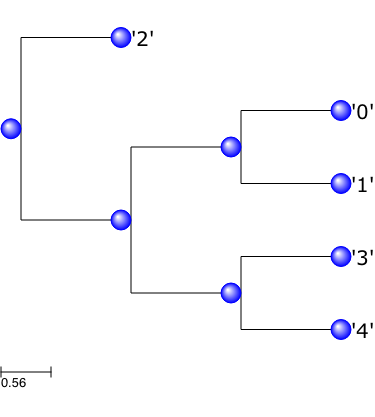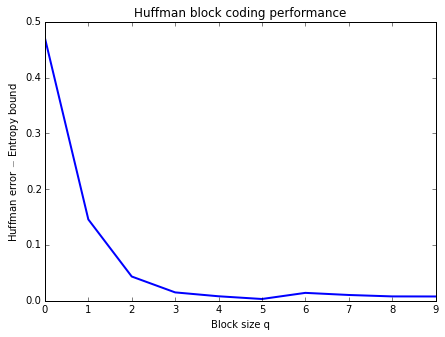# Entropic Coding and Compression¶

Important: Please read the installation page for details about how to install the toolboxes. $\newcommand{\dotp}{\langle #1, #2 \rangle}$ $\newcommand{\enscond}{\lbrace #1, #2 \rbrace}$ $\newcommand{\pd}{ \frac{ \partial #1}{\partial #2} }$ $\newcommand{\umin}{\underset{#1}{\min}\;}$ $\newcommand{\umax}{\underset{#1}{\max}\;}$ $\newcommand{\umin}{\underset{#1}{\min}\;}$ $\newcommand{\uargmin}{\underset{#1}{argmin}\;}$ $\newcommand{\norm}{\|#1\|}$ $\newcommand{\abs}{\left|#1\right|}$ $\newcommand{\choice}{ \left\{ \begin{array}{l} #1 \end{array} \right. }$ $\newcommand{\pa}{\left(#1\right)}$ $\newcommand{\diag}{{diag}\left( #1 \right)}$ $\newcommand{\qandq}{\quad\text{and}\quad}$ $\newcommand{\qwhereq}{\quad\text{where}\quad}$ $\newcommand{\qifq}{ \quad \text{if} \quad }$ $\newcommand{\qarrq}{ \quad \Longrightarrow \quad }$ $\newcommand{\ZZ}{\mathbb{Z}}$ $\newcommand{\CC}{\mathbb{C}}$ $\newcommand{\RR}{\mathbb{R}}$ $\newcommand{\EE}{\mathbb{E}}$ $\newcommand{\Zz}{\mathcal{Z}}$ $\newcommand{\Ww}{\mathcal{W}}$ $\newcommand{\Vv}{\mathcal{V}}$ $\newcommand{\Nn}{\mathcal{N}}$ $\newcommand{\NN}{\mathcal{N}}$ $\newcommand{\Hh}{\mathcal{H}}$ $\newcommand{\Bb}{\mathcal{B}}$ $\newcommand{\Ee}{\mathcal{E}}$ $\newcommand{\Cc}{\mathcal{C}}$ $\newcommand{\Gg}{\mathcal{G}}$ $\newcommand{\Ss}{\mathcal{S}}$ $\newcommand{\Pp}{\mathcal{P}}$ $\newcommand{\Ff}{\mathcal{F}}$ $\newcommand{\Xx}{\mathcal{X}}$ $\newcommand{\Mm}{\mathcal{M}}$ $\newcommand{\Ii}{\mathcal{I}}$ $\newcommand{\Dd}{\mathcal{D}}$ $\newcommand{\Ll}{\mathcal{L}}$ $\newcommand{\Tt}{\mathcal{T}}$ $\newcommand{\si}{\sigma}$ $\newcommand{\al}{\alpha}$ $\newcommand{\la}{\lambda}$ $\newcommand{\ga}{\gamma}$ $\newcommand{\Ga}{\Gamma}$ $\newcommand{\La}{\Lambda}$ $\newcommand{\si}{\sigma}$ $\newcommand{\Si}{\Sigma}$ $\newcommand{\be}{\beta}$ $\newcommand{\de}{\delta}$ $\newcommand{\De}{\Delta}$ $\newcommand{\phi}{\varphi}$ $\newcommand{\th}{\theta}$ $\newcommand{\om}{\omega}$ $\newcommand{\Om}{\Omega}$

This numerical tour studies source coding using entropic coders (Huffman and arithmetic).

In [ ]:
from __future__ import division

import numpy as np
import scipy as scp
import pylab as pyl
import matplotlib.pyplot as plt

from nt_toolbox.general import *
from nt_toolbox.signal import *

%matplotlib inline

In [ ]:



## Source Coding and Entropy¶

Entropic coding converts a vector $x$ of integers into a binary stream $y$. Entropic coding exploits the redundancies in the statistical distribution of the entries of $x$ to reduce as much as possible the size of $y$. The lower bound for the number of bits $p$ of $y$ is the Shannon bound :

$$p=-\sum_ih(i)\log_2(h(i))$$

where $h(i)$ is the probability of apparition of symbol $i$ in $x$.

Fist we generate a simple binary signal $x$ so that $0$ has a probability $p$ to appear in $x$.

Probability of 0.

In :
p = 0.1


Size.

In :
n = 512


Signal, should be with token 1,2.

In :
from numpy import random

x = (random.rand(n) > p) + 1


One can check the probabilities by computing the empirical histogram.

In :
h = [np.sum(x == 1), np.sum(x == 2)]
h = h/np.sum(h)

print("Empirical p = %.2f" %h)

Empirical p = 0.11


We can compute the entropy of the distribution represented as a vector $h$ of proability that should sum to 1. We take a max to avoid problems with null probabilties.

In :
e = - np.sum(h*np.log2([max(e,1e-20) for e in h]))
print("Entropy = %.2f" %e)

Entropy = 0.49


## Huffman Coding¶

A Hufman code $C$ associates to each symbol $i$ in $\{1,...,m\}$ a binary code $C_i$ whose length is as close as possible to the optimal bound $-\log_2\left(h(i)\right)$, where $h(i)$ is the probability of apparition of the symbol $i$.

We select a set of proabilities.

In :
h = [.1, .15, .4, .15, .2]


The tree $T$ contains the codes and is generated by an iterative algorithm. The initial "tree" is a collection of empty trees, pointing to the symbols numbers.

In :
m = len(h)
T =  * m # create an empty tree


We build iteratively the Huffman tree by grouping together the two erees that have the smallest probabilities. The merged tree has a probability which is the sum of the two selected probabilities.

Initial probability.

In :
#we use the symbols i = 0,1,2,3,4 (as strings) with the associated probabilities h(i)

for i in range(m):
T[i] = (h[i],str(i))


Iterative merging of the leading probabilities.

In :
while len(T) > 1:
T.sort() #sort according to the first values of the tuples (the probabilities)
t = tuple(T[:2])
q = T + T
T = T[2:] + [(q,t)]


We trim the computed tree by removing the probabilities.

In :
def trim(T):
T0 = T
if type(T0) == str:
return T0
else:
return (trim(T0),trim(T0))

T = trim(T)


We display T using the ete3 package (install it in the terminal with "pip install ete3").

In :
from ete3 import Tree, TreeStyle , NodeStyle, AttrFace, faces

t = Tree(str(T)+";")

ts = TreeStyle()
#ts.rotation = 90
ts.scale = 90
ts.branch_vertical_margin = 50 # 10 pixels between adjacent branches
ts.show_leaf_name = False #

nstyle = NodeStyle()
nstyle["shape"] = "sphere"
nstyle["size"] = 20
nstyle["fgcolor"] = "blue"

for n in t.traverse():
n.set_style(nstyle)

for node in t.iter_leaves():

t.render("%%inline", tree_style = ts)

Out:Once the tree $T$ is computed, one can compute the code $C_{i}$ associated to each symbol $i$. This requires to perform a deep first search in the tree and stop at each node.

In :
codes = {}

def huffman_gencode(T,codes,c):
if type(T) == str: #test if T is a leaf
codes[T] = c
else:
huffman_gencode(T,codes, c + "0")
huffman_gencode(T,codes, c + "1")

huffman_gencode(T,codes,"")


Display the code.

In :
for e in codes:
print("Code of token " + e + ": " + codes[e])

Code of token 1: 101
Code of token 3: 110
Code of token 0: 100
Code of token 2: 0
Code of token 4: 111


We draw a vector $x$ according to the distribution $h$.

Size of the signal.

In :
n = 1024


Randomization.

In :
from numpy import random

def rand_discr(p, m = 1):
"""
rand_discr - discrete random generator

y = rand_discr(p, n);

y is a random vector of length n drawn from
a variable X such that
p(i) = Prob( X=i )

"""

# makes sure it sums to 1
p = p/np.sum(p)

n = len(p)
coin = random.rand(m)
cumprob = np.append(0,+ np.cumsum(p))
sample = np.zeros(m)

for j in range(n):
ind = [(coin > cumprob[j]) & (coin <= cumprob[j+1])]
sample[ind] = j

return sample

x = rand_discr(h, n)


Exercise 1

Implement the coding of the vector $x$ to obtain a binary vector $y$, which corresponds to replacing each sybmol $x(i)$ by the code $C_{x(i)}$.

In :
run -i nt_solutions/coding_2_entropic/exo1

In :
## Insert your code here.


Compare the length of the code with the entropy bound.

In :
e = - np.sum(h*np.log2([max(e,1e-20) for e in h]))
print("Entropy bound = %.2f" %(n*e))
print("Huffman code  = %.2f" %len(y))

Entropy bound = 2197.95
Huffman code  = 2228.00


Decoding is more complicated, since it requires to iteratively parse the tree $T$.

Initial empty decoded stream.

In :
x1 = []


Perform decoding.

In :
T0 = T
for e in y:
if e == '0':
T0 = T0
else:
T0 = T0
if type(T0) == str:
i = i+1
x1 += T0
T0 = T


We test if the decoding is correct.

In :
from numpy import linalg

err = linalg.norm(np.subtract(x,[float(e) for e in x1]))
print("Error (should be zero) : %f " %err)

Error (should be zero) : 0.000000


## Huffman Block Coding¶

A Huffman coder is inefficient because it can distribute only an integer number of bit per symbol. In particular, distribution where one of the symbol has a large probability are not well coded using a Huffman code. This can be aleviated by replacing the set of $m$ symbols by $m^q$ symbols obtained by packing the symbols by blocks of $q$ (here we use $m=2$ for a binary alphabet). This breaks symbols with large probability into many symbols with smaller proablity, thus approaching the Shannon entropy bound.

Generate a binary vector with a high probability of having 1, so that the Huffman code is not very efficient (far from Shanon bound).

Proability of having 0.

In :
t = .12


Probability distriution.

In :
h = [t, 1-t]


Generate signal.

In :
from numpy import random

n = 4096*2
x = (random.rand(n) > t) + 1


For block of length $q=3$, create a new vector by coding each block with an integer in $\{1,...,m^q=2^3\}$. The new length of the vector is $n_1/q$ where $n_1=\lceil n/q\rceil q$.

Block size.

In :
q = 3


Maximum token value.

In :
m = 2


New size.

In :
n1 = (n//q+1)*q


New vector.

In :
x1 = np.zeros(n1)
x1[:len(x)] = x
x1[len(x):] = 1
x1 = x1 - 1
x2 = []

for i in range(0,n1,q):
mult = [m**j for j in range(q)]
x2.append(sum(x1[i:i+q]*mult))


We generate the probability table $H$ of $x_1$ that represents the probability of each new block symbols in $\{1,...,m^q\}$.

In :
H = h
for i in range(q-1):
Hold = H
H = []
for j in range(len(h)):
H = H + [e*h[j] for e in Hold]


A simpler way to compute this block-histogram is to use the Kronecker product.

In :
H = h
for i in range(1,q):
H = np.kron(H, h)


Exercise 2

For various values of block size $k$, Perform the Huffman coding and compute the length of the code. Compare with the entropy lower bound.

In :
run -i nt_solutions/coding_2_entropic/exo2

Entropy bound = 0.529361
---
Huffman(block size = 1) = 1.000122
Huffman(block size = 2) = 0.675171
Huffman(block size = 3) = 0.572876
Huffman(block size = 4) = 0.544434
Huffman(block size = 5) = 0.537476
Huffman(block size = 6) = 0.532593
Huffman(block size = 7) = 0.543579
Huffman(block size = 8) = 0.539795
Huffman(block size = 9) = 0.537231
Huffman(block size = 10) = 0.537109In :
## Insert your code here.